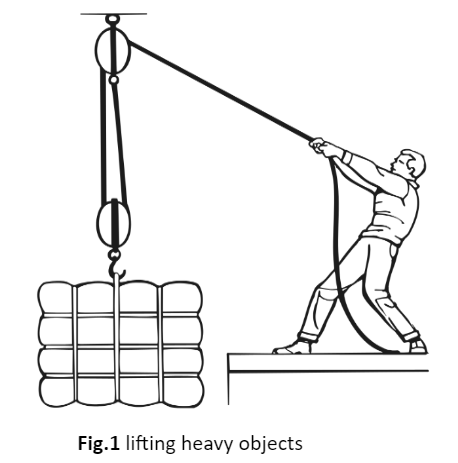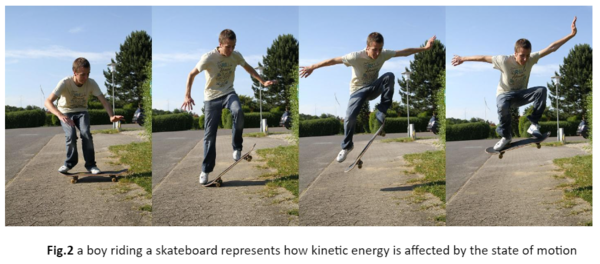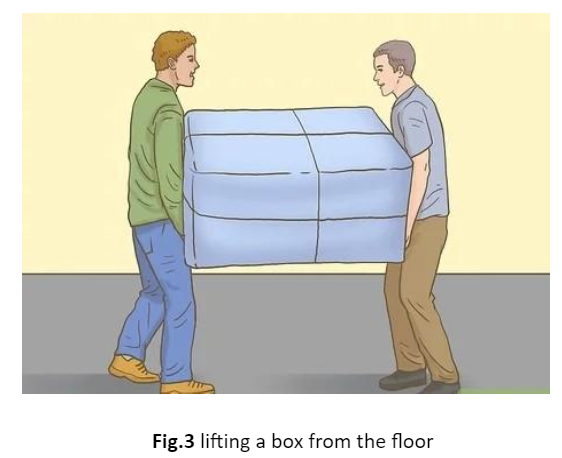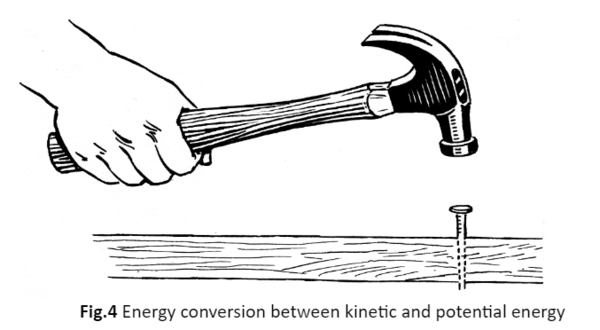• ### Browse All Lessons

##### Assign Lesson

Help Teaching subscribers can assign lessons to their students to review online!

 Tweet# Energy

This lesson aligns with Next Generation Science Standards (NGSS) PS2.A

Introduction
A boy pushes a table on a horizontal surface and moves it from the dining hall to the room; it causes a change. In this situation, the boy changes the position of the table. If we observe it scientifically, we would say that boy is doing work on the table. When an object is moved over a distance by an external force, then the work is done. Energy is always required to do work. Energy is a fundamental term that tends to be used a lot in everyday life.  We use energy to walk, move our cars along roads and boats through the water, cook food on the stove, etc. In this article, we will learn about the term energy, types of energy, and the law of conservation of energy.

Energy
The term energy refers to the “ability to do work or cause change.” In other words, energy can be defined as the strength to do any kind of physical activity. Energy is considered a quantitative property that can be transferred from one object to another object. Thus, work is a transfer of energy. For instance, when we lift an object against the earth’s gravitation, we do work on it. We use energy to lift that object from the ground.Unit of Energy
The S.I. unit used for the measurement of energy is “joule.” Energy is also expressed in other units such as ergs, calories, kilowatt-hours, and kilocalories.

Types of Energy
Many forms of energy can be grouped into two general types that are kinetic and potential.

Kinetic Energy
The word kinetic originates from the Greek word kinetos which means “moving.” Therefore, kinetic energy is directly associated with the object’s motion. The moving objects are capable of causing a change or are capable of doing work. The kinetic energy of moving objects depends upon both their mass and speed. Kinetic energy is determined by the following formula;For instance, if a boy riding a skateboard is at rest, the kinetic energy will be zero. As he moves the skateboard, the kinetic energy gradually starts increasing. Similarly, when the weight of the boy is caused to the high speed of the board, a large amount of kinetic energy is generated. We can say that kinetic energy increases as the mass increases, and it is also affected as the speed of the objects increases.Potential Energy
The objects can store energy due to their position and their shape that stored energy is known as potential energy. All energy has the same unit, which is the joule.

For example, when we lift a box from the floor, we change its position. In simple words, we transfer energy that is stored in it. Similarly, when we compress a spring, the energy we use to compress is also stored in it. The stored energy due to the position or shape of an object is considered potential energy. Mathematically, it can represent as;Energy Transformation and Conservation
When an object does work on another object, some of its energy transfers to the second object. The movement of energy from one place or object to another is known as energy transfer. One of the most common transformations is the transformation between the potential energy and the kinetic energy.

For instance, a person has a hammer in their hand. As they raise the hammer higher, the energy is stored in the form of potential energy. When they drop the hammer downwards to the nail on a table’s surface, the stored potential energy is converted into kinetic energy as the hammer falls. When the hammer hits the nail, then the kinetic energy is again transformed into potential energy, and now the stationary hammer will have potential energy.This example represents that energy is neither destroyed nor created during the whole process – it is only transformed from one form to another. This proves the law of conservation of energy. When the kinetic energy and potential energy of an object are combined, it is known as mechanical energy.

Summary
• Energy is defined as the ability of an object to perform work.
• All forms of energy can be categorized into two types that are kinetic energy and potential energy.
• Kinetic energy is the energy due to the motion of an object, e.g., a rollercoaster. it depends on the mass and speed of the moving objects.
• The energy stored due to the position or shape of an object is referred to as potential energy, e.g., the water on the top of waterfalls.
• Energy is neither destroyed nor created, it is only altered from one form to another.

Related Worksheets: